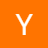### 什么是面条代码

if...if 型

```function demo (a, b, c) {
if (f(a, b, c)) {
if (g(a, b, c)) {      // ...
}    // ...
if (h(a, b, c)) {      // ...
}
}
if (j(a, b, c)) {    // ...
}
if (k(a, b, c)) {    // ...
}
}```if-if-before

else if...else if 型

```function demo (a, b, c) {
if (f(a, b, c)) {
if (g(a, b, c)) {      // ...
}    // ...
else if (h(a, b, c)) {      // ...
}    // ...
} else if (j(a, b, c)) {    // ...
} else if (k(a, b, c)) {    // ...
}
}```else-if-before

else if 最终只会走入其中的某一个分支，因此并不会出现上面组合爆炸的情形。但是，在深度嵌套时，复杂度同样不低。假设嵌套 3 层，每层存在 3 个 else if，那么这时就会出现 3 ^ 3 = 27 个出口。如果每种出口对应一种处理数据的方式，那么一个函数内封装这么多逻辑，也显然是违背单一职责原则的。并且，上述两种类型可以无缝组合，进一步增加复杂度，降低可读性。

### 基本情形if-if-after

### 查找表

```const rules = {
x: function (a, b, c) { /* ... */ },
y: function (a, b, c) { /* ... */ },
z: function (a, b, c) { /* ... */ }
}
function demo (a, b, c) {
const action = determineAction(a, b, c)
return rules[action](a, b, c)
}```else-if-lookup

### 职责链模式else-if-chain

```const rules = [
{    match: function (a, b, c) { /* ... */ },    action: function (a, b, c) { /* ... */ }
},
{    match: function (a, b, c) { /* ... */ },    action: function (a, b, c) { /* ... */ }
},
{    match: function (a, b, c) { /* ... */ },    action: function (a, b, c) { /* ... */ }
}  // ...]```

rules 中的每一项都具有 match 与 action 属性。这时我们可以将原有函数的 else if改写对职责链数组的遍历：

```function demo (a, b, c) {
for (let i = 0; i < rules.length; i++) {
if (rules[i].match(a, b, c)) {
return rules[i].action(a, b, c)
}
}
}```

### 总结

×

×

#### 评论列表 :共有2人吐槽, 3633人围观